﻿ Asymmetric supercapacitors based on high capacitance Ni<sub>6</sub>MnO<sub>8</sub> and graphene
 Chinese Chemical Letters2019, Vol. 30Issue (6): 1329-1334PDF
Asymmetric supercapacitors based on high capacitance Ni6MnO8 and graphene
Mingyang Zenga,1, Li Zhanga,*1, Dandan Wua, Jun Maa, Cao Zhanga, Shujuan Lib,*a Department of Physics, School of Science, Lanzhou University of Technology, Lanzhou 730050, China;
b Institute for Mathematics, Free University of Berlin, Berlin D-14195, Germany
Abstract: Supercapacitors with high energy density and high power density have attracted numerous attentions. Here, we successfully synthesized Ni6MnO8 material by a fast, facile, and cost-effective method, which the area specific capacitance was found to be as high as 1113 mF/cm2 at a current density of 1 mA/cm2. Further, the asymmetric supercapacitor was assembled by using Ni6MnO8 as the positive electrode and graphene as the negative electrode with an operation potential from 0 to 1.4 V. It exhibited an area specific capacitance of 69.1m F/cm2 at 0.5 mA/cm2 and stable cycling performance which presented about 80% capacitance retention after 5000 cycles at 5 mA/cm2. The energy density of graphene//Ni6MnO8 supercapacitors was calculated to be 18.81 mWh/cm2 at a power density of 350.1 mW/cm2 and still remained 5.8 mWh/cm2 at a power density of 6990.7 mW/cm2, meaning that the asymmetric supercapacitor combine excellent power density and energy density.
Keywords: Ni6MnO8     Electrode     Asymmetric supercapacitors     Power density     Energy density

At present, supercapacitors are widely used to electric vehicles, grid, mobile intelligent products, etc., due to their fast charge and discharge, high power density and long cycle lifetimes [1-3]. However, the energy density of supercapacitors is still unable to meet market requirements, which still restricts their further practical applications [4, 5]. Therefore, in the future, advanced supercapacitors with high energy density must be developed without sacrificing the power density and cycle life. It is known that energy density (E) can be improved by maximizing the specific capacitance (Cs) and/or the working voltage (V) according to the following equation : E = 1/2CsV2. Thus, there are two ways to improve the energy density of supercapacitors. One is to construct electrode materials which exhibit high specific capacitance. It is known that pseudocapacitive materials always show higher specific capacitance than double layer capacitive materials, but these materials usually suffer from shorter cyclic lifetimes . The other is to widen the potential window. Compared with the first way, widening potential window is the more effective because energy density E is proportional to the square of the voltage. Asymmetric supercapacitors are found to be effective alternatives to increase the energy density because they usually operate wider potential window and have the higher specific capacitance than symmetric supercapacitors [8, 9]. The asymmetric supercapacitors commonly consist of two different electrodes, which can make full use of the different potential windows of the two electrodes to provide a large operation voltage, accordingly resulting in high energy density [4, 10, 11].

In order to improve specific capacitance, various materials such as transition metal oxides, metal hydroxides, and conducting polymer materials have been investigated extensively in supercapacitors [7, 12-15]. Ni-Mn-O binary oxide is an attractive electrode material and widely used in various supercapacitors [16-18]. In all Ni-Mn-O binary oxides, Ni6MnO8 is one of the most promising positive electrode candidates for its high theoretical specific capacitance, low cost, abundant resources and environmental friendliness. More importantly, it is reported that Ni6MnO8 possesses better electrical conductivity, and higher electrochemical activity than that of nickel oxides or manganese oxide [19, 20]. Since both nickel and manganese ions can participate in the redox reactions, Ni6MnO8 is expected to offer richer redox sites [21-26].

On the other hand, carbon materials, exhibiting more complementary electrochemical windows compared with various pseudocapacitive materials such as Ni(OH)2 and cobalt oxides, are most widely used as negative electrodes in supercapacitors [5, 14, 27-30]. In various of carbon materials, graphene, with a unique two-dimension morphology, emerges as a promising negative electrode materials in supercapacitors due to its superb characteristics of chemical stability, high electrical conductivity and large surface area.

In this work, we successfully fabricated Ni6MnO8 materials by a fast, facile, and cost-effective method, and the area specific capacitance was calculated to be as high as 1113 mF/cm2 at a current density of 1 mA/cm2. Furthermore, we designed a new supercapacitor using Ni6MnO8 as the positive electrode and graphene as the negative electrode. The supercapacitors could cycle reversibly in a wide potential window of 0–1.4 V and exhibit stable cycling performance of about 80% capacitance retention after 5000 cycles at 5 mA/cm2. Meanwhile, the supercapacitor exhibited the area specific capacitance of69.1 mF/cm2 at 0.5 mA/cm2, and it also reached an energy density of 18.81 mWh/cm2 at a power density of 350.1 mW/cm2, still remaining 5.8 mWh/cm2 at a power density of 6990.7 mW/cm2.

Ni6MnO8 was fabricated through a hydrothermal method and subsequent anneal process. Before the experiment, Ni-foam (2 cm × 2 cm) was immersed in hydrochloric acid and ultrasonicated for 10–20min to remove oxide layer on the surface. In a typical procedure, 0.582 g of Ni(NO3)2·6H2O and 5 mL of Mn(NO3)2 were dissolved in a mixture of N-methyl pyrrolidone (NMP 55 mL) and H2O (5 mL). Then the solution and treated Ni-foamwere transferred to a 100 mL Teflon autoclave for hydrothermal reaction at 180 ℃ for 8 h. After cooling down to room temperature, the Ni-foam was collected and washed with deionized water and ethanol several times. After drying in oven at 60 ℃ for 12 h, the substrate was sintered to 400 ℃ for 2 h in air. Finally, the Ni-foam supported Ni6MnO8 was obtained. As the negative electrode materials, graphene was prepared by modified Hummers method as reported somewhere else . All the chemicals were analytical grade and used as received. The products were characterized using X-ray diffraction (XRD; Shimadzu XRD-6000), scanning electron microscopy (SEM, JSM 6701 F), transmission electron microscopy (TEM, JEOL 2100 FEG) and X-ray photoelectron spectra (XPS, ESCALAB250x).

In half-cell tests, the electrochemical tests were conducted in a three-electrode systemwith 2 mol/L KOH as the aqueous electrolyte. Platinum foil and Hg/HgO electrodes were used as counter and reference electrodes, respectively. The obtained Ni-foam supported Ni6MnO8 (2 cm × 2 cm) was firstly cut into four pieces of 1 cm × 1 cm quadrate shape and serves directly as the working electrode. Toverify the growth uniformity of 2 cm × 2 cm electrode, we fabricated three electrodeswith 2 cm × 2 cmNi-foam and measured the difference in mass of Ni-foam (2 cm × 2 cm) before and after the reaction. The loading mass of Ni6MnO8 active material in every electrode (~4 cm2 in area) was about 4 mg. Then these three 2 cm × 2 cm electrodes divided into four 1 cm × 1 cm electrodes, respectively. We measured the mass of each 1 cm × 1 cm electrode, which was roughly equal, indicating that the effect of mass equilibration on electrochemical performance can be neglected. In addition, to reduce the volume of the electrode, Ni-foam supported Ni6MnO8 was compressed at a pressure of 7 MPa before electrochemical measurement. In full cell tests, the asymmetric supercapacitor device was assembled into a coin cell to measure the performances. The Ni-foam supported Ni6MnO8 was used as the positive electrode and graphene served as the negative electrode. The negative electrode was prepared by the traditional slurry coating method. Graphene, acetylene black and polytetrafluoroethylene (PTFE) binders were mixed in a mass ration of 80:10:10 to obtain a homogeneously dispersed slurry. Then the slurry was drop-dried onto Ni-foam (1 cm × 1 cm). The as-prepared electrodeswere driedin avacuumoven at 60 ℃ for 12 h.TheNi-foam with sample loaded was compressed at a pressure of 7 MPa before electrochemical measurement.

All of the electrochemical measurements were carried out by using CHI660D electrochemical working station (Shanghai, China) at room temperature. Electrochemical performance of as-obtained electrodes was evaluated by cyclic voltammetry (CV), galvanostatic charge-discharge (GCD) and electrochemical impedance spectroscopy (EIS). The electrodes were first activated by several CV cycles until the CV curves remained stable. To assemble supercapacitors, the mass loading for the negative electrode was determined by balancing the charges stored in each electrode. According to the specific capacitance calculated from galvanostatic charge-discharge curves, the loading mass ratio of active materials (graphene//Ni6MnO8) was estimated to be 2:1.

Fig. 1a shows that the XRD pattern of Ni6MnO8 sample matches well with the cubic phase Ni6MnO8 crystalline structure (JCPDS No. 89-4619). The intense diffraction peaks at 2θ = 18.4°, 35.8°, 37.5°, 43.6° and 63.3° can be assigned to (111), (311), (222), (400) and (440), respectively. No characteristic peaks of impurities are detected indicting complete conversion from precursors to Ni6MnO8. The crystal structure of Ni6MnO8 can be seen in Fig. 1b, which is consisted of MnO6 and NiO6 octahedron. The morphology of the sample was examined by SEM as shown in Figs. 1c and d. It clearly shows that Ni6MnO8 nanosheets arrays are uniformly distributed on the Ni-foam substrate. Under higher magnification in Fig. 1d, Ni6MnO8 nanosheets are quite uniform with the thickness of 32 nm. Obviously, there is plenty of space between nanosheets, which is beneficial for electrolyte diffusion. Meanwhile, the larger accessible surface area can provide an important morphological foundation for ultrahigh specific capacitance. Further investigation of the crystalline structure of Ni6MnO8 was carried out in TEM. As shown in Figs. 1e and f, the nanosheets exhibit smooth surface and thin thickness. Under high resolution in Fig. 1f, the crystal lattice spacing is measured to be 0.208 nm, which corresponds well with the spacing between (400) planes in Ni6MnO8 cubic structure. The corresponding selected area electron diffraction (SAED) of Ni6MnO8 shows ring pattern, indicating the polycrystalline state of these nanosheets. The diffraction rings can be assigned to (311) and (400) planes of Ni6MnO8 phase, which is in good agreement with XRD measurement in Fig. 1a.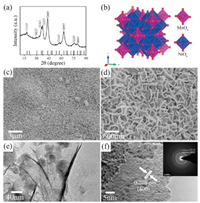Download: larger image Fig. 1. Structure of Ni6MnO8. (a) X-ray diffraction pattern of Ni6MnO8 (JCPDS No. 89-4619). (b) The crystal structure of Ni6MnO8. (c, d). SEM images of Ni6MnO8 sample at different magnifications. TEM image (e) and HRTEM (f) of Ni6MnO8.

To investigate the as-prepared Ni6MnO8 in detail, XPS study was carried out to determine the chemical composition and electronic structure. The XPS spectra of Ni6MnO8 is shown in Fig. 2a, which reveals the presence of Ni, Mn, O in Ni6MnO8. The C element peak located at 284.4 eV can be ascribed as carbon substrate employed in XPS. Fig. 2b exhibits the Ni 2p spectrum of Ni6MnO8, in which the main peak is located at 855.1, 861.8 and 873.4 eV corresponding to the state of Ni2+ [32, 33]. As shown in Fig. 2c, the two peaks located at 641.5 and 653.3 eV respectively, with an energy separation of 11.8, are in accordance with the reported data of Mn4+ in Ni6MnO8 . In Fig. 2d, the peaks of O 1s at 529.4 and 530.9 eV can be assigned to M–O-M or M–O (M = Ni, Mn) [35, 36].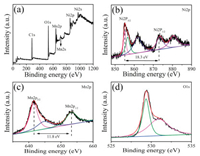Download: larger image Fig. 2. XPS survey spectrum (a) and high resolution XPS spectra of Ni 2p (b), Co 2p (c) and O 1s (d).

CV test was conducted to evaluate the electrochemical properties of Ni6MnO8. Fig. 3b shows the representative CV curves of the Ni6MnO8 electrode at various scan rates ranging from 5 mV/s to 200 mV/s. Obviously, redox peaks at –0.27 V and –0.38 V can be observed during the positive and negative voltage sweeps of CV curves in all scan rates, indicating that the electrochemical behavior of the Ni6MnO8 electrode is pseudocapacitive behavior. Pseudocapacitive behavior is distinct from electric double-layer capacitance characterized by nearly rectangular CV curves. The anodic peaks (at positive current density) and cathodic peaks (at negative current density) in the CV curves result from the oxidation and reduction processes of Ni6MnO8, respectively. This typical redox peaks may be assigned to the redox reaction M–O or M–OOH with the OH- ions in alkaline electrolyte (M represents Ni and Mn) [37, 38]. In addition, it is seen that the anodic and cathodic peaks shift to higher and lower potentials with increasing the scan rate, respectively, which may be due to the polarization. However, electrodes still preserve outstanding reversibility of redox reaction with offering a symmetric redox peaks when the scan rate rises to 100 mV/s. Besides, to investigate the contribution of current collector, the CV curve of pure Ni-foam was also recorded (Fig. 3a). Obviously the integrated area of CV curve of Ni-foam is much smaller than that of Ni6MnO8 material at a scan rate of 10 mV/s, where the specific capacitances of the Ni-foam and Ni6MnO8 material are 59.6 mF/cm2 and 1113 m F/cm2, respectively. Thus the contribution of Ni-foam is negligible.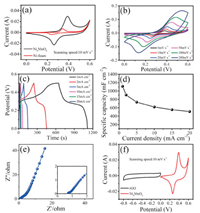Download: larger image Fig. 3. Electrochemical performance of Ni6MnO8. (a) Comparative CV curves of Ni-foam and Ni6MnO8. (b) CV curves of Ni6MnO8 at different scan rates. (c) Galvanostatic charge/discharge curves at various current densities. (d) Area specific capacity at various current densities. (e) Nyquist plots of the electrode in the frequency range of 100 kHz to 0.01 Hz. (f) Comparative CV curves of Ni6MnO8 and graphene electrodes. The CV curves are performed in 2.0 mol/L KOH aqueous electrolyte.

To further evaluate the electrochemical properties of the assynthesized materials as electrodes, GCD measurement was carried out at various current densities ranging from 1 mA/cm2 to 20 mA/cm2 (Fig. 3c). It is obviously that the platform in the charge-discharge indicates that the electrochemical behavior of the Ni6MnO8 electrode is pseudocapacitive in accordance with CV results. Furthermore, there are two separate plateaus in the charge or discharge process located at 0.3-0.4 V and 0.2-0.3 V, which demonstrate the redox processes of the active material while charging or discharging. Notably, the galvanostatic charge-discharge curves of Ni6MnO8 electrode are highly symmetrical without an obvious iR drop at low current densities, indicating a rapid current-voltage response and an excellent electrochemical reversibility.

The specific capacitance of the electrode can be calculated from the GCD curves according to the following equation: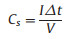(1)

where Cs is the area specific capacitance ( mF/cm2), I is the current density ( mA/cm2), Δt is the discharge time (s), and V indicates the voltage (V). The area specific capacities at various discharge current densities are plotted in Fig. 3d. The area specific capacities are found to be 1113, 906, 735, 620, 555 and 510 mF/cm2 at 1, 2, 5, 10, 15 and 20 mA/cm2, respectively. It can be seen that the area specific capacities gradually decrease with increasing the current density, which may be ascribed to the diffusion of electrolyte ions is limited because of the time constraint at high current density. Some electrolyte ions do not participate in the Faradaic redox reaction due to the slow ions diffusion and low electron transfer at higher current density. In fact, at high current densities, only the outer active surface was applied to the charge storage, so resulting in a lower electrochemical utilization of electroactive materials . Rate capability is an important indicator for the use of supercapacitors in power applications. A good electrochemical supercapacitor is required to provide high energy density at a high charge/discharge rate. The specific capacitance of Ni6MnO8 electrode material is 1113 mF/cm2 at 1 mA/cm2, and still 45.8% of the specific capacitance is retained when the discharge current density increases to 20 mA/cm2. This result means the excellent high-rate properties of Ni6MnO8.

EIS is a useful technique for evaluating the kinetic and mechanistic information of electrode materials, which was performed at 0.3 V from 100 kHz to 0.01 Hz with an AC amplitude of 5 mV. Nyquist plots are shown in Fig. 3e. In high frequency region, the intercept at real axis (Z') is about 0.7 Ω, representing a low equivalent series resistance (ESR). In addition, no obvious semicircle in the high frequency range can be observed, which indicates the charge-transfer resistance (Rct) aroused from Faradic reaction and electrochemical double layer capacitance is negligible. Therefore, the design of highly conductive Ni6MnO8 material grown on current collector can reduce the contact resistance between electrode material and current collector, and is favorable for high performance electrochemical application. The straight slope line at low frequency region is 2.6, which represents Warburg resistance (ZW) related to ion diffusion/ transport in the electrolyte [40, 41]. This further illustrates the reason for Ni6MnO8 having good electrochemical properties.

To further demonstrate the superior capacitive performance of Ni6MnO8 nanosheets on Ni-foam and to construct a supercapacitor with high energy density and high power density, an asymmetric supercapacitor cell was assembled using the Ni6MnO8 nanosheets and graphene as positive and negative electrodes, respectively. To further estimate the stable potential windows, CV curves of Ni6MnO8 and graphene were performed at a scan rate of 10 mV/s in 2 mol/L KOH aqueous solution (Fig. 3f). The Ni6MnO8 electrode was measured within a potential window of 0 to 0.6 V, while graphene was measured within a potential window of -0.8 V to 0 V (Fig. 3f). Noticeably, it can be observed that the two electrodes are quite stable in a different potential window. If the total cell voltage can be expressed as the sum of the potential range for Ni6MnO8 and for graphene, the potential of supercapacitors can be extended up to 1.4 V in 2 mol/L KOH aqueous solution.

For supercapacitors, it is well-known that the charge balance between the two electrodes will follow the relationship q+ = q-. Generally, the charges stored in positive and negative electrode can be determined by following Eq. (2) :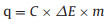(2)

where C represents the specific capacitance of positive electrode or negative electrode, ΔE is the potential range (V), m is the mass of the active material in electrode (g).

In order to obtain the charge balance q+ = q-, the mass balance will be expressed as follows: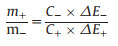(3)

Based on the above analysis of the specific capacitance values and potential ranges for the Ni6MnO8 and graphene, the optimal loading mass ratio of active materials (graphene//Ni6MnO8) was estimated to be 2:1 in the supercapacitors.

Fig. 4a exhibits the CV curves of the optimized supercapacitors at various scan rates from 10 mV/s to 1000mV/s. With increasing the scan rates, the shape becomes less rectangular, possibly because of diffusion limitations. However, when the sweep speed increases to 1000 mV/s, the rectangle remains, indicating thatgraphene//Ni6MnO8 supercapacitors have good rate performance. To further evaluate the capacitivepropertiesofthe supercapacitors, GCDmeasurementswere carried out at various current densities ranging from 0.5 mA/cm2 to 10 mA/cm2 (Fig. 4b). It can be clearly observed that all the curves are highly linear at various current densities, which is typical characteristic of an ideal supercapacitor. It can be observed that the discharge curve is nearly symmetric with its corresponding charging counterpart, demonstrating the excellent electrochemical reversibility and good coulombic efficiency. No obvious iR drop can be observed for any of the curves, meaning that the supercapacitors have low internal resistance. These all indicate that graphene//Ni6MnO8 supercapacitor was an ideal supercapacitor. The specific capacitance of graphene//Ni6MnO8 supercapacitor at different current densities is shown in Fig. 4c. The area specific capacities are 69.1, 57.6, 44.7, 30.6, 21.4 mF/cm2 at 0.5, 1, 2, 5, 10 mA/cm2, respectively. Obviously it can be seen that the specific capacitance gradually decreases with increasing current densities.This may be because diffusion of electrolyte ions was on account of the time constraint at high current densities, and only theouteractive surface canbeutilizedfor charge storage, resultingin a lower electrochemical utilization of electroactive materials.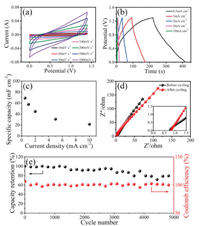Download: larger image Fig. 4. Electrochemical performance of supercapacitors. (a) CV curves of graphene//Ni6MnO8 supercapacitors in 2 mol/L KOH electrolyte at different scan rates. (b) Galvanostatic charge/discharge curves at different current densities of 0.5, 1, 2, 5, and 10 mA/cm2, respectively. (c) Area specific capacity of graphene//Ni6MnO8 supercapacitors at different current densities. (d) Electrochemical impedance spectroscopy of asymmetrical devices before and after 5000 cycles. (e) Cycle performance of graphene//Ni6MnO8 supercapacitors at a current density of 5 mA/cm2.

In addition, cycle stability also is an important performance indicator for supercapacitors. The cycling test was measured by the repeated charging-discharging at a current density of 5 mA/cm2 for 5000 cycles (Fig. 4e). The initial specific capacitance is as high as 69.1 mF/cm2 at the current density of 5 mA/cm2. It is observed that 96.8% of the initial specific capacitance can be recovered after 3000 cycles and 80% capacitance is still retained after 5000 cycles, which means that specific capacitance of graphene//Ni6MnO8 supercapa-citors maintains for numerous cycles without noticeable decrease. In the cycling, the coulombic efficiency of graphene//Ni6MnO8 supercapacitor is close to 100%, which also means that graphene//Ni6MnO8 supercapacitor demonstrated stable and outstanding cycle performance. The cycle performance was very competitive with other asymmetric supercapacitors, such as porous graphene//Ni(OH)2/graphene (94.3% retention after 3000 cycles) , activated carbon (AC)//NiCo2O4@MnO2 (71% retention after 5000 cycles)  and AC//LiNi0.5Mn1.5O4 (95% retention after 1000 cycles) .

For further understanding the graphene//Ni6MnO8 supercapacitors cycle process, electrochemical impedance spectroscopy of graphene//Ni6MnO8 supercapacitors before and after 5000 cycles was measured (Fig. 4d). It is clearly shown that the value of ESR is about 0.75 Ω before cycling and the value is 0.8 Ω after cycling without the obvious change. It is obvious that the Rct before cyclingis smaller than that of after cycling, indicating the charge-transfer resistance increases after the cycling process. This is probably due to the lossof activematerials on the current collector, and the corrosion of the current collector caused by electrolytes during the charge/ discharge processes. Before cycling, the slope of the curve in low frequency range is 1.3, resulting from the frequency dependence of ion diffusion/transport in the electrolyte. After 5000 cycles, an almost identical impedance curve is obtained, further demonstrating the long term electrochemical stabilityof theNi6MnO8 materials.

In addition, a good electrochemical supercapacitor is required to provide high energy density at a high charge/discharge rate. The power density and energy density are generally used as important parameters to characterize the electrochemical performance of electrochemical storage device. Energy density (E) is derived from the galvanostatic charge and discharge measurements using the following equation: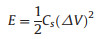(4)

where Cs is the area specific capacitance of the supercapacitors, and ΔV is the potential window. Power density (P) is calculated from the following equation: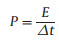(5)

where E is energy density and Δt is discharge time. The energy density of graphene//Ni6MnO8 supercapacitors calculated is found be 18.81 mWh/cm2 at a power density of 350.1 mW/cm2, and still remains 5.8 mWh/cm2 at a power density of 6990.7 mW/cm2. For convenience of comparisonwith other materials, we also measured and calculated mass energy density of supercapacitor, which corresponding to 16.51 Wh/kg at 374.74 W/kg and 6.4 Wh/kg at 7 kW/kg. Fig. 5 shows the Ragone plots of graphene//Ni6MnO8 supercapacitors at different current densities. The energy and power densities were evaluated from the galvanostatic discharge curves on the account of the total mass containing both electrode active materials. Compared with various other transitional metal oxides based asymmetric supercapacitors, our supercapacitor delivers a higher energy density than various Co oxides or Mn oxides based asymmetric supercapacitors, including (MEGO)//Co3O4@MnO2 (17.7 Wh/kg at 158 W/kg, and 3.3 Wh/kg at 8 kW/kg) , MnO2//AC (25.2 Wh/kg at 100 W/kg, and 6.9 Wh/kg at 2.5 kW/kg), NiCo2O4@MnO2//AC(35Wh/kg at 163 W/kg, and7.8Wh/kg at 1125 W/kg), Ni(OH)2/GNs/NF//AC (11.1 Wh/kg at 93 W/kg) . Such high energy density can be attributed to the high specific capacitance and the large cell voltage. Meanwhile, the power density of our supercapacitor is also higher than other ternary transition metal compound based asymmetric supercapacitors, such as graphene//CoMoO4(8.18 Wh/kg at 92 W/kg, and 6.875 Wh/ kg at 190 W/kg) .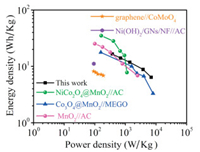Download: larger image Fig. 5. Ragone plots of the asymmetric graphene//Ni6MnO8, Co3O4@MnO2//MEGO, NiCo2O4@MnO2//AC, MnO2//AC, graphene//CoMoO4 and Ni(OH)2/GNs/NF//AC supercapacitor.

In summary, Ni6MnO8 nanosheets were prepared through a simple hydrothermal method and the annealing process. The area specific capacitance was found to be as high as 1113 mF/cm2 at current density of 1 mA/cm2. Furthermore, the asymmetric supercapacitor was assembled Ni6MnO8 as the positive electrode and graphene as the negative electrode. The supercapacitors could cycle reversibly in a wide potential window of 0–1.4 V and exhibit stable cycling performance which can present about 80% capacitance retention after 5000 cycles at 5 mA/cm2. In addition, the energy density of graphene//Ni6MnO8 supercapacitors was calculated be 18.81 mWh/cm2 at a power density of 350.1 mW/cm2, and still remained 5.8 mWh/cm2 at a power density of 6990.7 mW/cm2. Such supercapacitors offer great promise for the application of high-performance energy storage systems because of the advantages of simple fabrication process and excellent electrochemical performance.

Acknowledgments

This work was supported by the Scientific Research Foundation of the Higher Education Institutions of Gansu Province (No. 2015A-037), the Natural Science Foundation of Gansu Province (No. 1506 RJZA095), and China Postdoctoral Science Foundation.

References